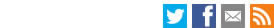Search the LSE website# Abstract:CEP Discussion Paper
Aggregation and the Gravity Equation
Stephen J. Redding and David E. Weinstein
January 2019
Paper No' CEPDP1595:
Full PaperJEL Classification: F10; F14; C43

One of the most successful empirical relationships in international trade is the gravity equation, which relates bilateral trade flows between an origin and destination to bilateral trade frictions, origin characteristics, and destination characteristics. A key decision for researchers in estimating this relationship is the level of aggregation, since the gravity equation is log linear, whereas aggregation involves summing the level rather than the log level of trade flows. In this paper, we derive an exact Jensen’s inequality correction term for the gravity equation in a nested constant elasticity of substitution (CES) import demand system, such that a log-linear gravity equation holds exactly for each nest of this demand system. We use this result to decompose the effect of distance on bilateral trade in the aggregate gravity equation into the contribution of a number of different terms from sectoral gravity equations. We show that changes in sectoral composition make a quantitatively relevant contribution towards the aggregate effect of distance, particularly for more disaggregated definitions of sectors.